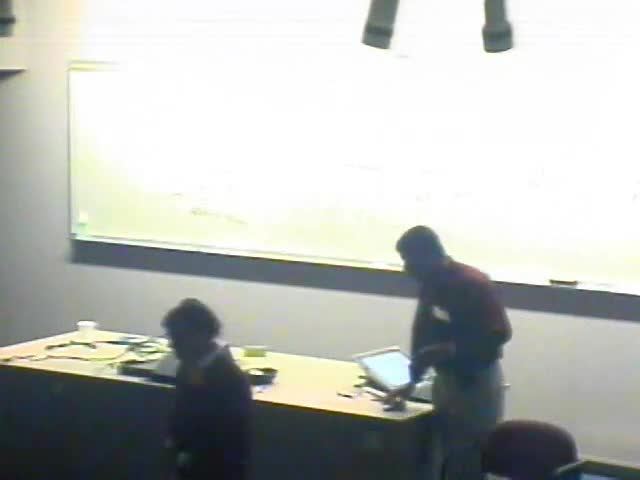### Videos

#### Statistical Analysis of Shapes of 2D Curves, 3D Curves, and Facial Surfaces

##### Presenter
April 4, 2006
Keywords:
• Statistical Models
MSC:
• 60K35##### Abstract
Our previous work developed techniques for computing geodesics on shape spaces of planar closed curves, first with and later without restrictions to arc-length parameterizations. Using tangent principal component analysis (TPCA), we have imposed probability models on these spaces and have used them in Bayesian shape estimation and classification of objects in images. Extending these ideas to 3D problems, I will present a "path-straightening" approach for computing geodesics between closed curves in R3. The basic idea is to define a space of such closed curves, initialize a path between the given two curves, and iteratively straighten it using the gradient of an energy whose critical points are geodesics. This computation of geodesics between 3D curves helps analyze shapes of facial surfaces as follows. Using level sets of smooth functions, we represent any surface as an indexed collection of facial curves. We compare any two facial surfaces by registering their facial curves, and by comparing shapes of corresponding curves. Note that these facial curves are not necessarily planar, and require tools for analyzing shapes of 3D curve. (This work is in collaboration with E. Klassen, C. Samir, and M. Daoudi)